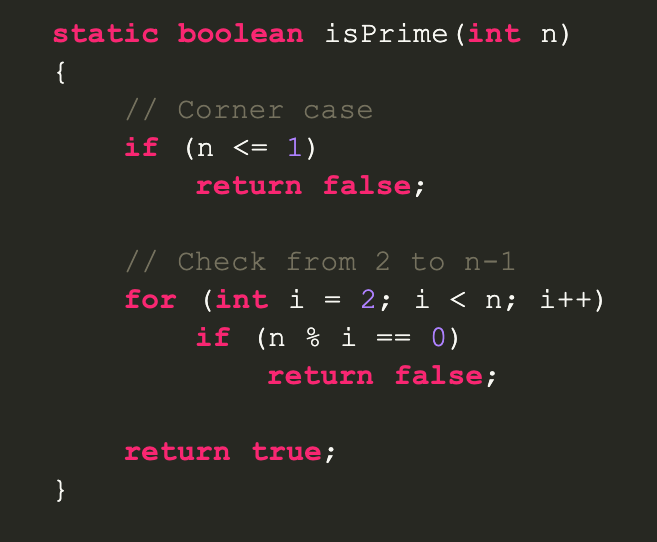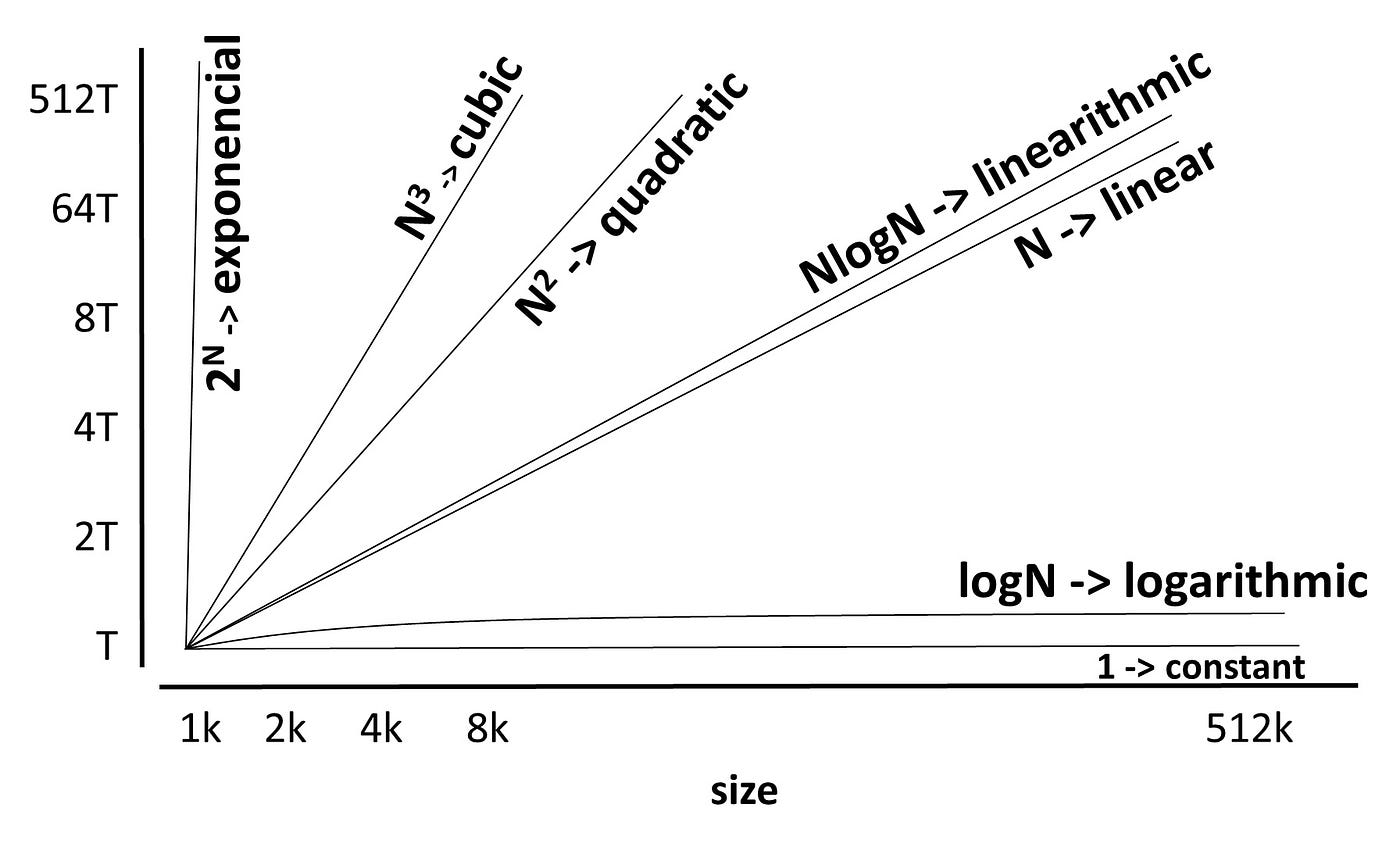# Big O Notation: Calculate Time & Space ComplexityIn order to check if a number is prime we will loop through all numbers from 2 to but excluding the actual number and check its divisibility.As an algorithm grows in size its execution time can be expected to increase. Unless in the case of constants, these usually involve simple calculations without loops.
`public class Add{  public static void main(String[] args)  {    int num1 = 8; // initialize num1 to be 8    int num2 = 4; // initialize num2 to be 4    System.out.println(num1 + num2); // Output should be 12  }}// Don't worry if you don't know Java syntax just know we are getting the sum of the two numbers`
`public class NestedLoop{  public static void main(String[] args)  {    int x = 0; // This will increment and serve as the outer counter    int y = 5; // This will decrement and serve as the inner counter        while (x < 10)    {      System.out.println("Outer counter: " + x);      while (y > 0)      {        System.out.println("Inner counter: " + y);        y = y - 1;      }      x = x + 1;    }    System.out.println("Loop Completed.");}`